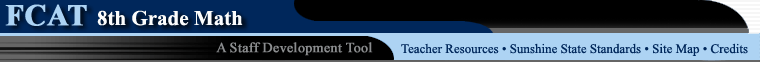Welcome to the Eighth Grade Math Staff Development Tool. Click on the links below to view video clips of model math lessons in real classrooms. Each lesson is accompanied by teacher plans and student worksheets. For even more lessons and other materials, visit the Math Resources section.Lesson: Drops on a Penny: Graphing DataLesson: Get a Half Life: Exponential EquationsLesson: Go Fish: Solving ProportionsLesson: Exploring Linear Equations: Y-Intercept and SlopeLesson: Hands-On Equations: One- and Two-Step EquationsLesson: Shaquille O'Neal: Exploring RatiosLesson: Spaghetti Bridges: Linear EquationsMath Resources: Lesson Plans, Student Worksheets, Notes, and More! Send comments to: winkelma@usf.edu Return to FCIT HomeProduced by the Florida Center for Instructional Technology
College of Education, University of South Florida ©2013-2013